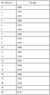# Millikan Oil Drop Experiment: Determining Elementary Charge

• Sandro Romualdez

## Homework Statement

Calculate the charge on each oil drop and determine the elementary charge on an electron given the following:
Voltage (Attached)
d (Distance between two charged plates) = 0.10m
m (Of the droplet) = 1.57x10^-15 kg
g = 9.8 kgm/s^2

q = mg*d / ΔV

## The Attempt at a Solution

I'm just confused on how I should first tackle the problem.

Should I just calculate the ΔV between each voltage, and use that in the equation to calculate the charge between each test/zap (The charge on each drop?), and then get the average charge of all using the mean formula? (Sum of q's/20)

Thanks for any help.

#### Attachments

•Capture.PNG
3.6 KB · Views: 751